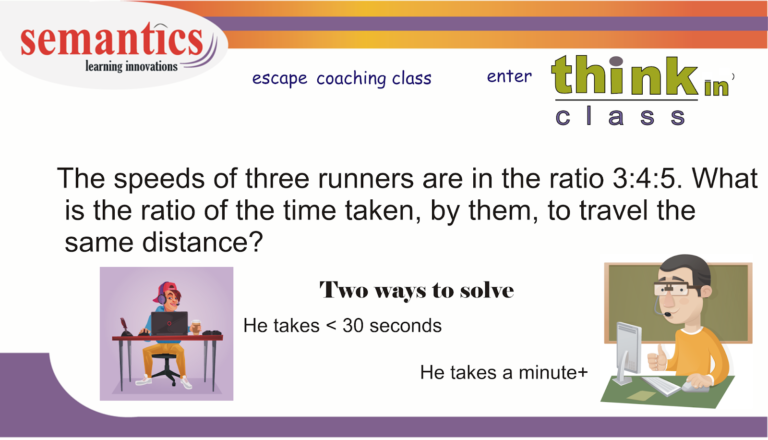# Math problem solving skills 2There are two ways to solve this problem

### Approach 1 :This approach would require you to assume variables,write equations and solve them.

Yes! this approach take some time

Those of you who are new to math, would, most likely, attempt a question using this approach

First :
Convert the ratio into number by introducing a constant x

The speed of the 3 runners will be 3x,4x and 5x.

Second
Assume the distance traveled by each runner is 60 units ( 60 is the LCM of 3,4,5. You can take any number.. I took the LCM to avoid decimals.)

Speed = distance/time. Hence Time = distance/speed

Time will be 60/3x,60/4x,60/5x. respectively = 20/x,15/x,12/x

Remove (1/x) and then you can write the numbers in a ratio: 20:15:12

### Approach 2:If you solve a mathematical question using this approach, it means you are comfortable

• determining the nature of numbers,
• identifying relationships between variables
• substituting values to arrive at an answer quickly

This approach would take less time than approach 1.

Now lets solve this question using this approach

You would have observed the following in the question ratio of the speeds are given distance is constant

The relationship between speed and time is : Speed is inversely proportional to time.

Hence if speed increases time decreases and vice versa.

The speeds are in the ratio 3:4:5.

Hence the times should be in the ratio: 1/3 :1/4: 1/5 as speed is inversely proportional to time.

Multiplying with LCM (4,3,5) i.e. 60 …………..to normalize the ratio

We get 20: 15:12

### Take-away:Always observe the hidden relationship between numbers

Hope you understood both the methods.

Need math help: Get in touch with me: